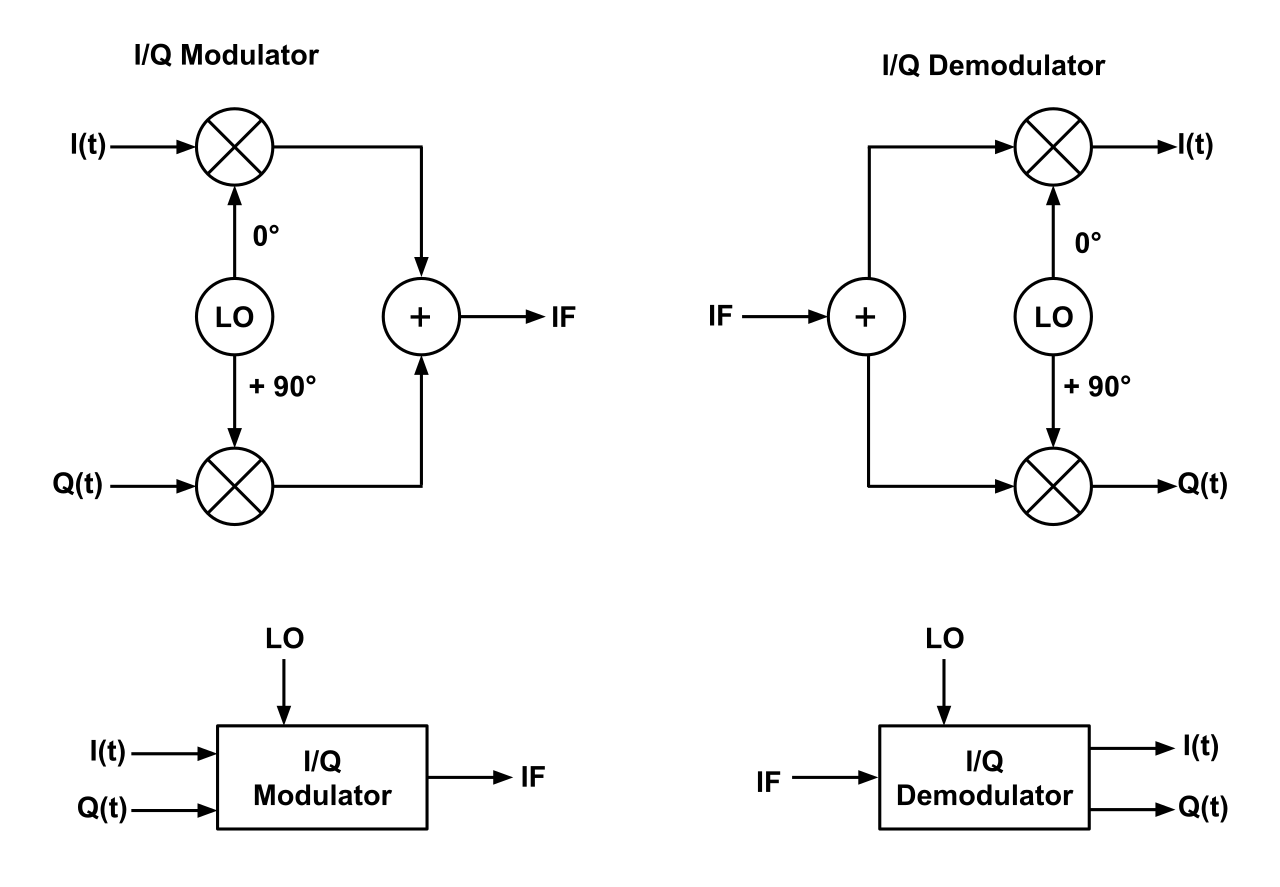# IQ Signals and IQ Modulation/Demodulation

The following application note provides an introduction to IQ signals and modulation.

## 1. On IQ Signals

A complex wave can be represented using two orthogonal value pairs, in the form of IQ signals. These two orthogonal numbers represent a complex waveform with a 90° phase relationship. The $$I$$ stands for “in-phase” and $$Q$$ stands for “quadrature”. In fact, a consine and sine wave are quadrature waveforms of each other.

We can say mathematically that our quadrature signal is represented as $$I*cos(2\pi f t)$$ and $$Q*sin(2\pi f t)$$, where by convention, the $$I$$ is the amplitude of the in-phase and $$Q$$ is the amplitude of the quadrature components. Figure 1 below shows this:

##### Figure 1: Basic IQ Signal (With I=Q=1)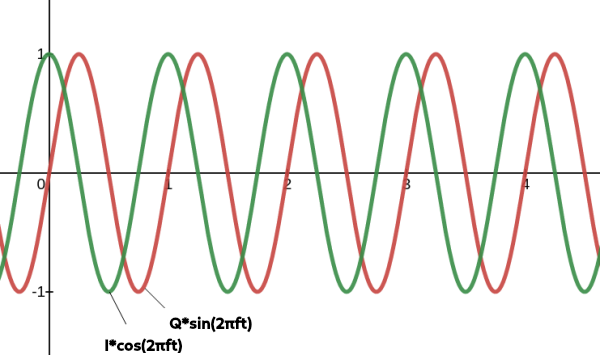### 1.1 Basics of IQ Modulation/Demodulation

The key to IQ modulation and demodulation is how we add quadrature signals for various modulation schemes. The block diagram below shows how adding $$I$$ and $$Q$$ works;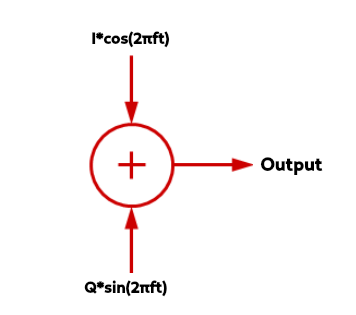As an example, by simply adding the I and Q values together, by superposition, if we were to say $$I=1$$ and $$Q=1$$, then we’d have a new waveform in black shown below:

##### Figure 3: Adding the I=1 and Q=1 Signal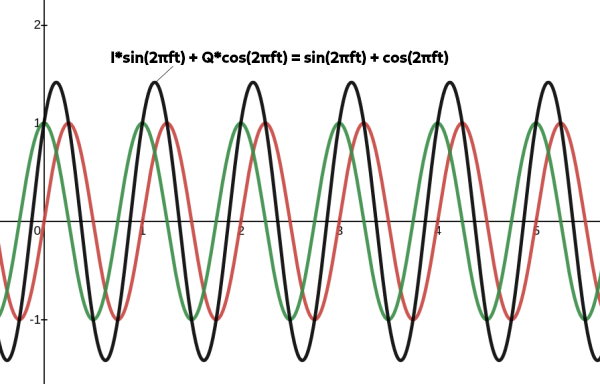##### Figure 3: Adding the I=1 and Q=3 Signal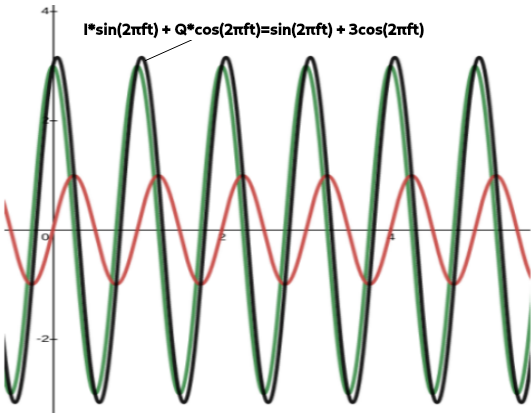In general, if $$I$$ or $$Q$$ changes or varies, the result are changes in amplitude, phase and frequency modulation of the sum. Essentially as the $$I$$ and $$Q$$ vectors change, the magnitude of the vector sum changes. The GIF below shows how this works using a phasor diagram– another way to visualize IQ signals:

##### Figure 5: How Adding I (x-axis) and Q (y-axis) Changes with a Phasor Diagram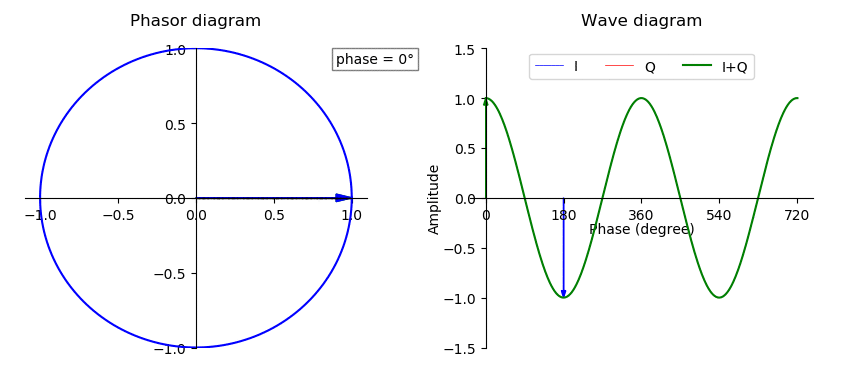Now, knowing how the complex plane works, and making our IQ signal a function of time, we can see that IQ pairs can be represented by a famous formula, Euler’s formula:

$${e}^{i2\pi f t}= cos(2\pi f t) + isin(2 \pi f t)$$

$$= I(t) + iQ(t)$$

And thus, we say the amplitude vector’s magnitude is given by:

$$A= \sqrt{(I^2 + Q^2)}$$

And the angle $$\theta$$ between the I vector and the A vector is:

$$\theta= tan^{-1}(Q/I)$$

### 1.2 IQ Modulators/Demodulators

To control how the $$I$$ and $$Q$$ signals are modulated/demodulated, the use of a IQ modulator and IQ demodulator is needed, which essentially mixes an LO frequency from 0-90° with the $$I(t)$$ and $$Q(t)$$ signals, as shown in the following block diagrams:

##### Figure 6: IQ Modulator and Demodulator